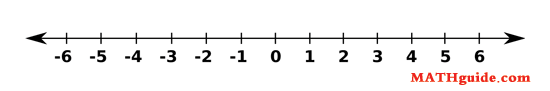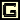Operations on Integers
 Home > Lessons > Operations on Integers Updated August 9th, 2017
Introduction

There are three appealing ways to understand how to add integers. We can use movement, temperature and money. Lastly, we will take a look at the rules for addition.
• Movement

You are probably familiar with a number line (see below). Traditionally, zero is placed in the center. Positive numbers extend to the right of zero and negative numbers extend to the left of zero. In order to add positive and negative integers, we will imagine that we are moving along a number line.Example 1:
If asked to add 4 and 3, we would start by moving to the number 4 on the number line -- exactly four units to the right of zero. Then we would move three units to the right. Since we landed up seven units to the right of zero as a result of these movements, the answer must be 7.

Example 2:
If asked to add 8 and -2, we would start by moving eight units to the right of zero. Then we would move two units left from there because negative numbers make us move to the left side of the number line. Since our last position is six units to the right of zero, the answer is 6.

Example 3:
If asked to add -13 and 4, we start by moving thirteen units to the left of zero. Then we move four units to the right. Since we land up nine units to the left of zero, the answer is -9.

Example 4:
If asked to add -6 and -5, first move six units to the left of zero. Then move five units further left. Since we are a total of eleven units left of zero, the answer is -11.

Here is a video that explains how to use a number line to add and subtract numbers.ideo: Add & Subtract Positive & Negative Numbers with Number Lines

• Temperature

The temperature model for adding integers is exactly the same as the movement model because most thermometers are really number lines that stand upright. The numbers can be thought of as temperature changes. Positive numbers make the temperature indicator rise. Negative numbers make the temperature indicator fall.

Adding two positive temperatures will result in a positive temperature, similar to example 1 above. Adding two negative temperatures will result in a negative temperature, similar to example 4 above.

Examples 2 and 3 can be understood in a different way by imagining a battle between two temperatures. When we added 8 and -2 in example 2, there was more positive temperature than negative temperature which would explain the result -- positive 6. In example 3 there was more negative temperature than positive. That will explain why the answer is negative.

• Money

It can be helpful to think of money when doing integer addition. The positive numbers represent income while the negative numbers represent debt.

When adding two incomes, like example 1 above, the answer has to be a bigger income and the result is a positive number. When adding two debts, like example 4 above, the answer has to be another debt. In fact, accountants would call it 'falling deeper in debt.'

Similar to our temperature battle between warm temperatures and cold temperatures, adding positive and negative numbers is like comparing income to debt. If there is more income than debt the answer will be positive, like example 2. If there is more debt than income the answer will be negative, like example 3.

Below is a table to help condense the rules for addition. Note the second and third rows of the body of the table. Those answers are dependant upon the original values.

 Rules for Addition Positive + Positive Positive Positive + Negative Depends Negative + Positive Depends Negative + Negative Negativeideo: Adding Positive and Negative Integersuizmaster: Adding Integersame: Pyramid Game: Addition

Instead of coming up with a new method for explaining how to subtract integers, let us borrow from the explanation above under the addition of integers. We will learn how to transform subtraction problems into addition problems.

The technique for changing subtraction problems into addition problems is extremely mechanical. There are two steps:

2. Take the opposite of the number that immediately follows the newly placed addition sign.

Let's take a look at example 1:

3 - 4

According to step #1, we have to change the subtraction sign to an addition sign. According to step #2, we have to take the opposite of 4, which is -4. Therefore the problem becomes:

3 + (-4)

Here are a few other examples:

Example 2: -2 - 8 = -2 + (-8) = -10

Example 3: 6 - (-20) = 6 + 20 = 26

Example 4: -7 - (-1) = -7 + 1 = -6uizmaster: Subtracting Integers

 Rules for Multiplication Positive x Positive Positive Positive x Negative Negative Negative x Positive Negative Negative x Negative Positive

Now we have to understand the rules. The first rule is the easiest to remember because we learned it so long ago. Working with positive numbers under multiplication always yeilds positive answers. However, the last three rules are a bit more challenging to understand.

The second and third steps can be explained simultaneously. This is because numbers can be multiplied in any order. -3 x 7 has the same answer as 7 x -3, which is always true for all integers. [This property has a special name in mathematics. It is called the commutative property.] For us, this means the second and third rules are equivalent.

One reason why mathematics has so much value is because its usefulness is derived from its consistency. It behaves with strict regularity. This is no accident, mind you. This is quite purposeful.

Keeping this in mind, let's take a look at Figure 2a below. There is a definite pattern to the problems in the table. The values in the first column remain constant but the values in the second column are decreasing by one, each step down the table. Consequently, the answer column is changing. The answers to each problem have a definite pattern as we go down the table too. It should be relatively easy to determine the two missing answers.

 Figure 2a 2 x 3 = 6 2 x 2 = 4 2 x 1 = 2 2 x 0 = 0 2 x -1 = ? 2 x -2 = ?

If you understand the pattern, you will see that the values in the answer column are decreasing. Each value in the answer column is two less than the value above it. See Figure 2b to see the solutions to those last two problems.

 Figure 2b 2 x 3 = 6 2 x 2 = 4 2 x 1 = 2 2 x 0 = 0 2 x -1 = -2 2 x -2 = -4

This should provide some meaning why a positive number times a negative number is always a negative number. Since we can multiply numbers in any order, it also explains why a negative number times a positive number is always a negative number.

5 x -6 = -6 x 5 = -30

Now, let us turn our attention to Figure 3a below. This table has a pattern similar to the one in Figure 2a. However, Figure 3a begins with a negative number in the first column. As we scan the list of answers in the last column, we can see that the last two problems remain unanswered.

 Figure 3a -8 x 3 = -24 -8 x 2 = -16 -8 x 1 = -8 -8 x 0 = 0 -8 x -1 = ? -8 x -2 = ?

With a little concentration, we can see that the two unanswered questions must have positive answers to maintain mathematical consistency. Why? The pattern within the last column shows that the values are increasing by 8 as we travel down the column. Figure 3b shows the table completely filled in.

 Figure 3b -8 x 3 = -24 -8 x 2 = -16 -8 x 1 = -8 -8 x 0 = 0 -8 x -1 = 8 -8 x -2 = 16

This should help us understand why a negative number times a negative number is always a postive number.

Here are some examples:

Example 1: 4 x -8 = -32

Example 2: -6 x 8 = -48

Example 3: -20 x -3 = 60ideo: Multiplying Positive and Negative Integersuizmaster: Multiplying Integersame: Pyramid Game: Multiplication

The rules for division are exactly the same as those for multiplication. If we were to take the rules for multiplication and change the multiplication signs to division signs, we would have an accurate set of rules for division.

Here are three examples:

Example 1: -9 ÷ 3 = -3

Example 2: 20 ÷ (-4) = -5

Example 3: -18 ÷ (-3) = 6uizmaster: Dividing Integers

Use these videos to solidify your knowledge of the skills and concepts presented within this section of MATHguide:ideo: Add & Subtract Positive & Negative Numbers with Number Linesideo: Adding Positive and Negative Integersideo: Multiplying Positive and Negative Integers

After reading the lessons, try our quizmasters. MATHguide has developed numerous testing and checking programs to solidify these skills:uizmaster: Adding Integersuizmaster: Subtracting Integersuizmaster: Multiplying Integersuizmaster: Dividing Integers

Use these games to have fun learning how to work with operations on Integers.ame: Pyramid Game: Additioname: Pyramid Game: Multiplicationame: Tic-Tac-Addition

Try this related lesson.esson: Divisibility